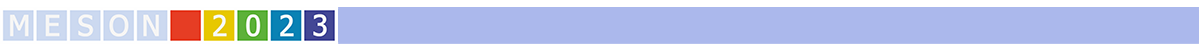#MESON2023

Jun 22 – 27, 2023
Auditorium Maximum
Europe/Warsaw timezone

## Physics Beyond the Standard Model with NA62

Jun 22, 2023, 4:05 PM
20m
Medium lecture hall (B) (Auditorium Maximum)

Parallel

### Speaker

Tomas Blazek (Comenius University Bratislava)

### Description

The NA62 experiment at CERN took data in 2016–2018 with the main goal of measuring the $K^+ \rightarrow \pi^+ \nu \bar\nu$ decay. The NA62 dataset is also exploited to search for light feebly interacting particles produced in kaon decays. Searches for $K^+\rightarrow e^+ N$, $K^+ \rightarrow \mu^+ N$ and $K^+ \rightarrow \mu^+ \nu X$ decays, where N and X are massive invisible particles, are performed by NA62. The N particle is assumed to be a heavy neutral lepton, and the results are expressed as upper limits of $O(10^{-8})$ of the neutrino mixing parameter $|U_{\mu 4}|^2$. The X particle is considered a scalar or vector hidden sector mediator decaying to an invisible final state. Upper limits of the decay branching fraction for X masses in the range 10–370 MeV/c$^2$ are reported. An improved upper limit of $1.0 \times 10^{-6}$ is established at 90$\%$ CL on the $K^+ \rightarrow \mu^+ \nu \nu \nu$ branching fraction.
Dedicated trigger lines were employed to collect di-lepton final states, which allowed establishing stringent upper limits on the rates lepton flavor and lepton number violating kaon decays. Upper limits on the rates of several $K^+$ decays violating lepton flavour and lepton number conservation, obtained by analysing this dataset, are presented.
The NA62 experiment can be run as a "beam-dump experiment" by removing the Kaon production target and moving the upstream collimators into a "closed" position. More than $10^{17}$ protons on target have been collected in this way during a week-long data-taking campaign in 2021. New results from analysis of this data, with a particular emphasis on Dark Photon and Axion-like particle Models, are reported.

Collaboration NA62

### Primary author

Angela Romano (University of Birmingham)